All submissions of the EM system will be redirected to Online Manuscript Submission System. Authors are requested to submit articles directly to Online Manuscript Submission System of respective journal.

# Review

, Volume: 9( 1) DOI: 10.37532/23206756.2021.9(1).203

## The Benards Convective Triggering Mechanism for the Origin of the Universe

*Correspondence:
Xingwu Xu
Research Institute of Hefei Gotion High-tech Power Energy Co; Ltd. Hefei, Anhui 230012, P. R. China
Tel: +86-551-62100917
E-Mail: xuxingwu@gotion.com.cn

Received: January 04, 2021; Accepted: January 14, 2021; Published: January 28, 2021

Citation: Xu X. The Benard’s Convective Triggering Mechanism for the Origin of the Universe. 2021;9(1):203.

### Abstract

Despite the great success of standard cosmology so far, it still has problems with the “initial singularity” and the Big Bang triggering mechanism. The “emergent cosmological model” is attracting more and more attention because it can phenomenogically solve the puzzle of initial singularity. In this model, the “singularity” is replaced by the “very small static micro-universe”. This micro-universe as well as the vacuum environment can be considered a dissipative system. Bernard’s convective cell is the typical feature of the dissipative system. This paper analyzes in detail each physical quantities corresponding to Bernard’s convective cell in the micro-universe. The conclusion is that the unevenness of matter density can lead to the “Bernard’s convective cell”, which will trigger the Big Bang. The important thing is that it is a spontaneous process. The tropical cyclone-like morphology of the spiral galaxies provides strong supporting evidence for the theory put forward by this paper. Moreover, these spiral galaxies have very similar rotation curve and rotation periods. This can be considered a “same gene” in these spiral galaxies.

### Keywords

The origin of the universe; Emergent universe; Bénard’s convective cell; Dissipative system

### Introduction

The current standard cosmology has achieved great success in many aspects. However, there are some serious problems in this model, such as “the problem of the primordial singularity of the universe” , “the issue of the start point of time” , “the triggering mechanism of the Big Bang” , etc. As one of the theoretical solutions to avoid the original singularity problem, the “emergent cosmological model” has attracted increasing attention [4-7]. The “emergent cosmological model” was put forward by Ellis and Maartens in 2004 . This model argues that the universe originated from a static micro-universe, then entering the inflation phase of standard cosmology. Here the “static universe” is just Einstein’s non-expansion static universe. The cosmological constant is absorbed in the potential energy V and the kinetic energy vanishes. The so-called “micro-universe” means that the scale-factor of the universe can be expressed as :(1)

When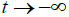the scale of the universe does not tend to zero, but a small enough scale 0 a which is larger than Plank scale. In this way, the primary singularity problem can be avoided. However, how does the transition from the static universe to the inflation phase occur? This model does not give an explanation. Therefore, the origin of the universe remains unsolved.

Benard’s convective self-organization triggering mechanism

Chakraborty considers that the primordial universe can be considered as the dissipative system . The dissipative system formed by the micro-universe and the vacuum environment is illustrated in FIG.1.

Figure 1: Schematic diagram of the dissipative system formed by primordial microuniverse and the vacuum environment.

Therefore, the system should have all properties of the dissipative system. As we have known, the typical feature of the dissipative system is the B nard’s convective cell phenomenon, which is shown in FIG. 2. and FIG.3.

Regarding the origin of the universe, in the frame of the dissipative system, the Big Bang must be a self-organizing process. The theoretical analysis of the self-organizing Big Bang is given below.

First of all, the matter in the primordial static micro-universe can be considered as a “fluid”. On the one hand, there is the exchange of matter and energy between the micro-universe and the vacuum environment, on the other, there must be a concentration difference in its own density distribution due to random fluctuations. There are two typical scenarios:

(1) Due to the random fluctuation, the concentration difference of matter density distribution is in the inner of the micro-universe, as

Figure 4: There are lower matter density areas and higher density areas in the micro-universe.

(2) Because the matter density of the primordial micro-universe is very high, it can be considered as a black hole. There is a possibility of the inhomogeneous density distribution between the horizon surface and the inner of the micro-universe (FIG. 5), which will lead to the surface tension gradient and “temperature gradient”.

Figure 5: The inhomogeneous density distribution between the horizon surface and the inner of the micro-universe.

Now let’s analyze the quantities that trigger rotation. For the fluid system, the three equations of hydrostatics are ,

i. The equation of continuity: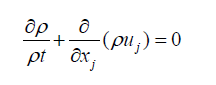(2)

Here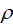is the matter density of the primordial micro-universe, xj (j = 1, 2, 3) denotes Cartesian coordinates, uj the velocity component.

ii. The equation of motion:(3)

Here Xi(i = 1, 2, 3) denotes the external force component (e.g. the interaction force between the micro-universe and the vacuum environment, the generalized gravity caused by the inhomogeneous matter density distribution),the stress (e.g. the surface tension gradient.), μ the coefficient of viscosity. The equation gives the equilibrium relation of each force.

iii. The equation of energy: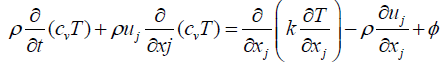(4)

Here cv is the specific heat at constant volume, T is temperature, Φ the dissipative energy generated from viscosity. Equation 4 indicates that the temperature gradient is the important driving force for the formation of B nard’s convective cell.

By means of Boussinesq approximation and the perturbation theory (first-order), equation 2 and equation 3 can be simplified to :(5)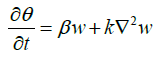(6)

Here w is the velocity, g the acceleration of gravity α, the cubic expansion coefficient θ, the perturbation of infinitely-small temperature,denotes the kinetic viscosity, β the temperature gradient,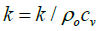denotes the coefficient of heat conduct. The Rayleigh number can be obtained from the solution of equation 5 and equation 6:(7)

Here g is the acceleration of gravity, d is the thickness of the liquid layer. In some situations, the temperature difference can be replaced by the concentration difference. By borrowing the result of reference  the Rayleigh number becomes:(8)

The meaning of each symbol can be found in reference . The definition of Grashof number (Gr) which reflects the relation between inertial force and viscous force is ,(9)

Therefore, RaGr reflects the competitive relation between convection and conduction, buoyancy and viscous forces. When Ra ≪ 1, Gr ≪ 1, heat conduct prevails. In the case of Ra ≫ 1, Gr ≪ 1,convection prevails, this is where the Benard’s convective cell is formed. For scenario , a concentration difference Δc in formula  must exist inside the primordial micro-universe. For the acceleration of gravity (��), we can use the generalized acceleration of gravity (ggr) to replace it. Therefore, the Rayleigh number becomes: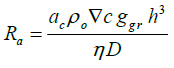(10)

Since the primordial micro-universe is a highly metastable system, its viscosity coefficient should be very small. Therefore, a very small concentration perturbation Δc will result in a very large Rayleigh number Ra, which will lead to convection cell (rotation). This is the origin of self-organizing rotation motion. For scenario , the cellular convection is caused by surface tension. The buoyancy term is not considered in the fluid heat conduction equation and motion equation, a Marangoni number can be obtained in the solutions of these equations [14,15],(11)

Here,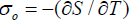denotes the rate at which entropy changes with temperature. As shown in FIG.6 there is a shallow, low-density on the surface of the horizon of the primordial micro-universe, the uneven distribution of matter density also leads to surface tension gradient and “temperature gradient”. When the Marangoni number is large enough the convective cell (rotation) can also be formed. Benard’s convective cell phenomenon is common in meteorology, which can further evolve into a powerful tropical cyclone . FIG.6.

In the primordial micro-universe, due to the density perturbation caused by random fluctuation, thus the B nard’s convective cell forms spontaneously, which further be amplified step by step and eventually forms the tropical cyclone. This is the Big Bang

Observational evidences

About the B nard’s convective cell triggering mechanism for the origin of the universe, the photos of spiral galaxies provide strong supporting evidence, which shows the morphology of tropical cyclone.

About the formation mechanism of the spiral galaxies, Eggen et al put forward a theory of “Tidal moment” : around 1 billion years ago, the angular momentum due to the tidal moments during the densification of adjacent galaxies results in rotation of galaxies. However, this theory is no longer widely accepted.

In fact, the origin of galaxies must be closely related to the origin of the universe. For the origin of the universe, the explanation of the standard cosmology is that the Big Bang is triggered by primordial density perturbation caused by quantum fluctuation, which is the “seeds” of the structure formation (galaxies, texture). But the theory cannot explain why the ratio of the spiral galaxies is so high. The spiral galaxy is rather popular galaxy in our universe.

According to the statistics of reference , 56% of galaxies are spiral galaxies. The morphology of these spiral galaxies is similar to the tropical cyclones. Moreover, the rotation curve of stars in these galaxies is similar, the rotation period is also similar . According to the reference , the rotation period of the Large Magellanic galaxy is around 0.25 billion years, which is surprisingly close to the Milky Way’s rotation period.

Regarding the formation and stability of the spiral arms in the spiral galaxies, the density wave theory was put forward by Lin & Shu in 1964 . In the theory, there is an important hypothesis that there is a “spiral gravitational field” in the spiral galaxies. However, this kind of “spiral gravitational field” can only be formed in the whirlpool Big Bang. These observational evidences show that the formation of the spiral galaxies carries a distinct spiral “close relatives” gene, which can be considered as the supporting evidence for the theory of “B nard’s convective triggering mechanism of the Big Bang.”

### Conclusion

Modern science permeates each other so that the inter-discipline sciences become more and more important. The combination of cosmology and astronomy is a major feature of this paper. In cosmology, “the origin of the universe” is still a problem worth exploring . Two pending issues left over by the standard cosmology are: “the singularity of the primordial universe” and “the triggering mechanism of the Big Bang”. According to emergency cosmology, the primordial universe is a static micro-universe with a nonzero scale. In this way, the primordial singularity can be avoid in phenomenological meaning. In this model, the static micro-universe and its vacuum environment constitutes a dissipative system. For a dissipative system, the Benard’s convective cell is a common phenomenon. In this paper, the physical quantities of Benard’s convective cell in the dissipative system are investigated in detail. The Rayleigh number ���� which reflects the competitive relationship between “convection flow” and “diffusion flow”, has a great relationship with the difference of matter density concentration Δc and the viscosity coefficient η in the static micro-universe. So that a very small perturbation of density will lead to a very large Rayleigh number, which will lead to the formation of the Benard convective cell. The surface tension of the primordial micro-universe also has this effect. And the spontaneous formation of the Benard’s convective cell is just the root cause of the Big Bang. In conjunction with the standard cosmology, what follows is the inflation of the universe and the formation of galaxies. The whirlpool explosion is bound to affect the formation of galaxies. Its deep mechanism needs further investigation. However, this “mother” gene must be passed on to galaxies. As observational evidence, the “tropical cyclone-like” morphology of spiral galaxies, the similar rotation curves of spiral galaxies, and the similar rotation periods fully reflect this “kinship”. Meanwhile, the whirlpool explosion must have an important effect on the gravitational field. If it can be proved that the “spiral gravitational field” comes from the whirlpool explosion, there will be a new explanation for the spiral galaxy arms formation and stabilization. Further, there will be a new interpretation of the dark matter. This will be the subject of further study.

### References

Welcome Message

Select your language of interest to view the total content in your interested language

###### Citations : 272

Journal of Physics & Astronomy received 272 citations as per Google Scholar report

#### Indexed In

• Open J Gate
• China National Knowledge Infrastructure (CNKI)
• Cosmos IF
• Directory of Research Journal Indexing (DRJI)
• MIAR
• Secret Search Engine Labs
• Euro Pub
• clarivate-web-of-science-logo-vector
• ICMJE

View More

### 9th International Conference on Physics

Edinburgh, Scotland

### 6th International Conference on Astronomy, Astrophysics and Space Science

Budapest, Hungary

Berlin, Germany

### 9th International Conference on Physics

Edinburgh, Scotland

### 6th International Conference on Astronomy, Astrophysics and Space Science

Budapest, Hungary

### 10th International Conference on Theoretical and Applied Physics